Courses

# Test: Cannizzaro Reaction

## 23 Questions MCQ Test Chemistry Class 12 | Test: Cannizzaro Reaction

Description
This mock test of Test: Cannizzaro Reaction for JEE helps you for every JEE entrance exam. This contains 23 Multiple Choice Questions for JEE Test: Cannizzaro Reaction (mcq) to study with solutions a complete question bank. The solved questions answers in this Test: Cannizzaro Reaction quiz give you a good mix of easy questions and tough questions. JEE students definitely take this Test: Cannizzaro Reaction exercise for a better result in the exam. You can find other Test: Cannizzaro Reaction extra questions, long questions & short questions for JEE on EduRev as well by searching above.
QUESTION: 1

### Only One Option Correct Type Direction (Q. Nos. 1-10) This section contains 10 multiple choice questions. Each question has four choices (a), (b), (c) and (d), out of which ONLY ONE is correct. Q.  The major organic product in the following reaction is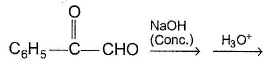Solution: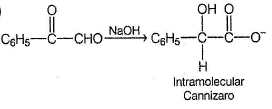QUESTION: 2

### Consider the following reaction,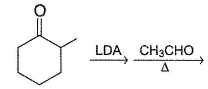Solution: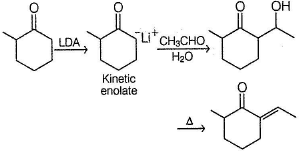QUESTION: 3

### Which is the best hydride (H-) donor in the key step of Cannizaro reaction?

Solution:

Dianion is better hydride donor. Also the electron donating group (CH3O) increases hydride (H-) donating a bility .

QUESTION: 4

Consider the following reaction,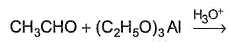Q.

The major organic product is

Solution:

It is a simple Tischenko reaction

QUESTION: 5

What is the major organic product in the following reaction?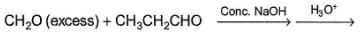Solution: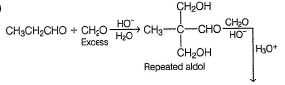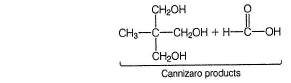QUESTION: 6

Predict the major organic product in the following reaction.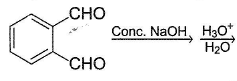Solution:

Intramolecular Cannizaro reaction occur.

QUESTION: 7

Whta is the major organic product formed in the follwing reaction ?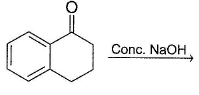Solution: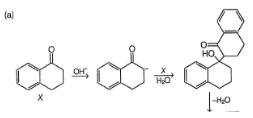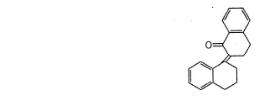QUESTION: 8

For a Cannizaro reaction,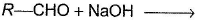Rate law is derived as : Rate = k [RCHO]2 [HO-]2
From the above rate law, it can be concluded that

Solution:

For formation of dianion hydride donor, an additional mole of NaOH is consumed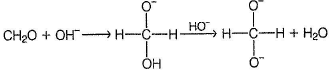Hence, rate becomes 2nd order with respect to HO- and fourth order overall.

QUESTION: 9

Consider the following reaction,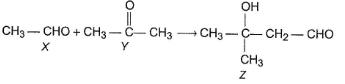Q.

How the product Z can be prepared selectively using X and Y and other reagents?

Solution: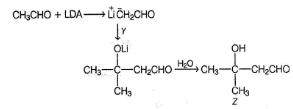QUESTION: 10

What is the major product formed in the following intramolecular Cannizaro reaction?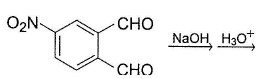Solution:

—CHO para to electron withdrawing —NO2 group is better hydride (H-) acceptor.

*Multiple options can be correct
QUESTION: 11

One or More than One Options Correct Type

Direction (Q. Nos. 11-15) This section contains 5 multiple choice questions. Each question has four choices (a), (b), (c) and (d), out of which ONE or MORE THAN ONE are correct.

Consider the cross Cannizaro reaction given below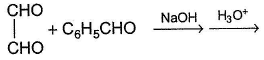Q.

The expected product(s) is/are

Solution: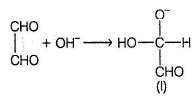(I) is formed by nucleophilic attack in the first step. (I) may undergo intramolecular hydride transfer giving option (a) or may undergo intermolecuiar hydride transfer to C6H5CHO giving option (c) and option (d).

*Multiple options can be correct
QUESTION: 12

In the Cannizaro reaction mentioned below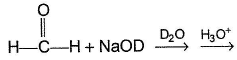Q.

The possible product(s) is/ar

Solution: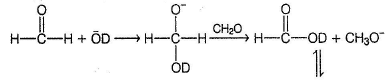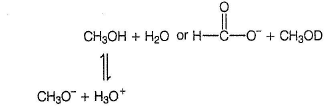*Multiple options can be correct
QUESTION: 13

What is/are the expected organic product(s) in the following reaction?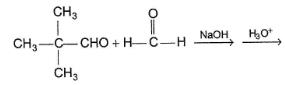Solution:

It is a case of cross Cannizaro reaction in which formaldehyde is always oxidised and the other aldehyde is reduced. So, correct option B and D.

*Multiple options can be correct
QUESTION: 14

Consider the following reaction,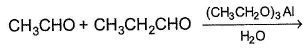Q.

The expected organic product(s) is/are

Solution:

It is a case of cross Tischenko reaction.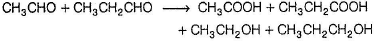Here, both can be oxidised or both can be reduced. In the next step ester formation occur by all possible combination of acid and alcohols.

*Multiple options can be correct
QUESTION: 15

Consider the following reaction sequence,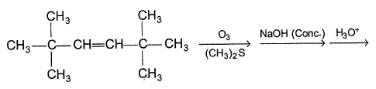Q.

The expected organic product(s) is/are

Solution: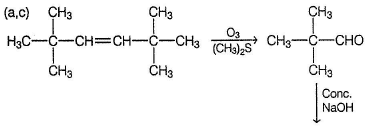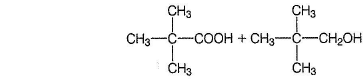QUESTION: 16

Comprehension Type

Direction (Q. Nos. 16-18) This section contains a paragraph, describing theory, experiments, data, etc.
Three questions related to the paragraph have been given. Each question has only one correct answer mong the four given options (a), (b), (c) and (d).

Passage

When aldehydes lacking a-hydrogen is treated with concentrated solution of a strong base, Cannizaro reaction takes place. In this reaction, one molecule of aldehyde is oxidised while other is reduced. The widely accepted mechanism is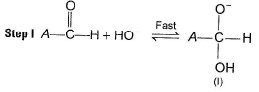Step II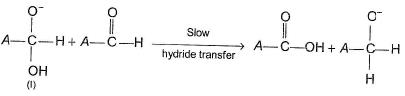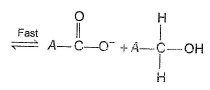Q.

What is the rate law derived from the above mechanism?

Solution: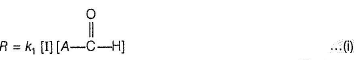The intermediate “I” can be determined as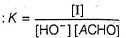Substituting [I] in Eq (i) gives
R = k1 K [ACHO]2 [HO-] = k [ACHO]2 [OH-']

QUESTION: 17

When aldehydes lacking a-hydrogen is treated with concentrated solution of a strong base, Cannizaro reaction takes place. In this reaction, one molecule of aldehyde is oxidised while other is reduced. The widely accepted mechanism isStep IIQ.

Which of the following observed fact establishes the correctness of the above mechanism?

Solution:

When reaction is carried out in D,O , no C—D bond formation occur. It establishes the correctness of above mechanism that reaction proceed by H- (hydride) transfer in the slow rate determining step.

QUESTION: 18

When aldehydes lacking a-hydrogen is treated with concentrated solution of a strong base, Cannizaro reaction takes place. In this reaction, one molecule of aldehyde is oxidised while other is reduced. The widely accepted mechanism isStep IIQ.

In certain experim ental condition, rate is found to be proportional to square of concentration of base (OH-).This indicates that

Solution:

Formation of dianion involves two moles of HO- per mole of aldehyde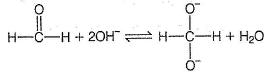QUESTION: 19

Matching List Type

Direction (Q. Nos. 19 and 20) Choices for the correct combination of elements from Column I and Column II are given as options (a), (b), (c) and (d), out of which one is correct.

Q.

Consider the reactions of Column I and match with the products of Column II. Mark the correct option from the codes given below.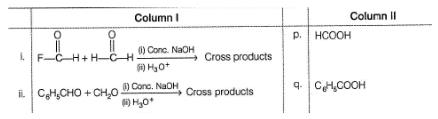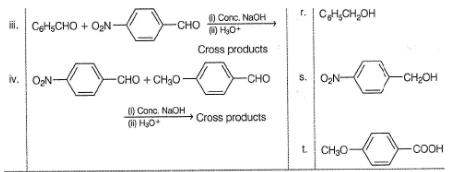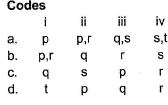Solution:

(i)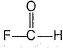is better H- acceptor, reduced to FCH2OH.
(ii) CH2O is oxidised and C6H5CHO is reduced in cross Cannizaro reaction.
(iii) Electron withdrawing —NO2 makes —CHO a better hydride acceptor, hence p-nitrobenzaldehyde is reduced and benzaldehyde is oxidised.
(iv) p-nitrobenzaldehyde is reduced, p-methoxy benzaldehyde becomes better hydride donor after attack by HO-, hence oxidised.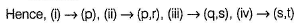QUESTION: 20

Match the reactions of Column I with the type of reactions from Column II. Mark the correct option from the codes given below.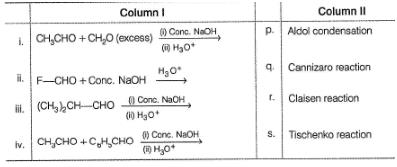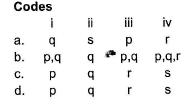Solution:

(i) Initially, aldol reaction followed by Cannizaro reaction giving C(CH2OH)4 + HCOOH.
(ii) F—CHO undergo Cannizaro reaction due to absence of α -H.
(iii) It has difficulty in aldol condensation, hence undergo Cannizaro reaction predominantly.
(iv) All aldol, Cannizaro and Claisen reaction occur.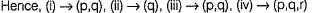*Answer can only contain numeric values
QUESTION: 21

One Integer Value Correct Type

Direction (Q. Nos. 21-23) This section contains 3 questions. When worked out will result in an integer from 0 to 9 (both inclusive).

Consider the following Cannizaro reaction,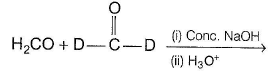Q.

How many different products are formed?

Solution:

Possible products are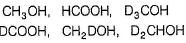*Answer can only contain numeric values
QUESTION: 22

Consider the following Cannizaro reaction,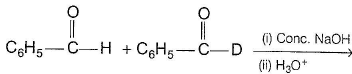Q.

How many different products are expected in this reaction?

Solution:

Possible products are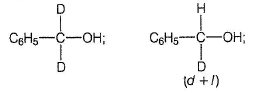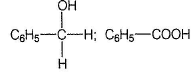*Answer can only contain numeric values
QUESTION: 23

Consider the following reaction,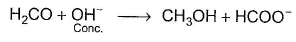Q.

If CH3OH is formed exclusively by hydride transfer from a dianion intermediat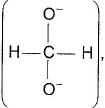what is the overall order of reaction?

Solution:

If dianion is hydride donor, the rate law is
R = k [H2CO]2 [OH-]2
Hence, reaction is overall fourth order.